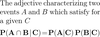Level 9 Level 11
Level 10

Ignore words

Check the boxes below to ignore/unignore words, then click save at the bottom. Ignored words will never appear in any learning session.

Ignore?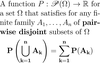Probability distribution (countable)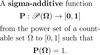(Probability) Distribution of a random variable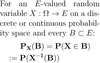PMF of a random variableCumulative distribution function (of a random variable)Conditional probability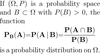Independent events (conditional)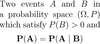Joint distribution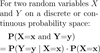Marginal distributionIndependent random variables (definition)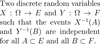Independent random variables (formula)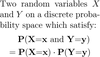Convolution (discrete)Conditionally independent